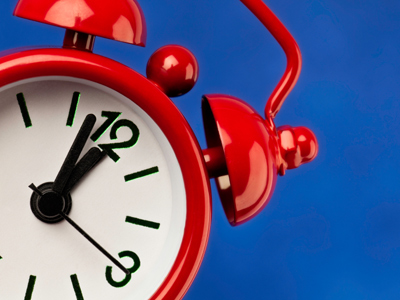When both hands are pointing to the 12, it's 12 o'clock.

# Year 1 Time - Telling the Time

This quiz addresses the requirements of the National Curriculum KS1 Maths and Numeracy for children aged 5 and 6 in year 1. Specifically this quiz is aimed at the section dealing with telling the time to the hour and to half past.

The first experience young children have of telling the time is usually when they learn how to say what hour it is (one o'clock, two o'clock and so on) by recognising the longer minute hand is pointing to the 12. This knowledge of telling the time gradually extends to recognising that when the minute hand points to the 6, it is half past the hour (half past one, half past two and so on).

To see a larger image, click on the picture.
Question 1
When it is half past, the longer minute hand points to which number?
12
6
4
8
The minute hand points straight down for half past
Question 2
What time does this clock show?
5 o'clock
4 o'clock
12 o'clock
8 o'clock
The hour hand is pointing to the 8
Question 3
What time does this clock show?
Half past 7
Half past 9
Half past 6
Half past 8
The hour hand is half way between the 8 and the 9, so it is halfway between 8 o'clock and 9 o'clock
Question 4
What time does this clock show?
8 o'clock
6 o'clock
1 o'clock
12 o'clock
When both hands point to the 12, it is 12 o'clock
Question 5
When it's 'o'clock', the longer hand is pointing to which number?
12
6
2
1
The minute hand must point to the 12 for it to be something o'clock. The shorter hand points to the hour
Question 6
What time does this clock show?
Half past 6
Half past 3
Half past 1
Half past 5
The minute hand is pointing to the 6 so it's half past. The hour hand is halfway between 1 and 2
Question 7
What time does this clock show?
3 o'clock
1 o'clock
12 o'clock
4 o'clock
The hour hand shows us that it's 1 o'clock
Question 8
What time does this clock show?
Half past 6
Half past 7
Half past 8
Half past 9
The hour hand is halfway between 7 and 8
Question 9
What time does this clock show?
12 o'clock
9 o'clock
2 o'clock
10 o'clock
The minute hand is pointing to the 12 and the hour hand is pointing to the 9
Question 10
What time does this clock show?
Half past 4
Half past 6
Half past 3
Half past 7
The minute hand is pointing straight down so it's half past. The hour hand is between the 6 and the 7